• 电机控制中，使用最多的就是PI控制器了，因为D参数通常使用不多。我打算以PMSM双环控制为例，对传统的PI控制进行分析。 下面是看到的一段PI控制器的介绍 按偏差的比例（P）、积分（I）和微分（D）进行控制的PID控制...
前言
电机控制中，使用最多的就是PI控制器了，因为D参数通常使用不多。我打算以PMSM双环控制为例，对传统的PI控制进行分析。
下面是看到的一段PI控制器的介绍

按偏差的比例（P）、积分（I）和微分（D）进行控制的PID控制器（亦称PID调节器）是应用最为广泛的一种自动控制器。它具有原理简单，易于实现，适用面广，控制参数相互独立，参数的选定比较简单等优点；而且在理论上可以证明，对于过程控制的典型对象──“一阶滞后+纯滞后”与“二阶滞后+纯滞后”的控制对象，PID控制器是一种最优控制。PID调节规律是连续系统动态品质校正的一种有效方法，它的参数整定方式简便，结构改变灵活（PI、PD、…）。

PI控制器具有一定的鲁棒性，因为它本身的定义上看，可以对误差校正，且校正本身不依赖于电机参数，只是PI控制器自身参数不合适的情况下，PI调节的动态性会不满意，最终稳态值可能也会有震荡。
PI控制器因为用的较多，参数设计方法很成熟。在使用FOC控制电机的情况下，电拖书里的直流电机双环参数设计方法可以被用于永磁同步电机的参数设计。当然，各家厂商对PI控制器的参数设计都提出过相应的设计方法，我个人是比较倾向于使用它们的设计方法，毕竟电拖书中要求PI控制器设计为I型和II型系统时，需要已知系统本身的延迟时间等参数，而这些参数比较难获得，厂商提供的电流环设计是按照一阶惯性环节设计的，还是比较简单的。
芯片厂商方案PI控制设计
ST的双环设计
电流环设计
设计时首先考虑进行电流环参数设计。当电机处于中低速运行时，可以忽略电流环输出的电压耦合项，电机的电流环PI参数配置，应当将PI调节器与电机构成一个闭环系统来看。
假设现在反电势电压为常数（因为通常相对于电流来说反电势电压变化缓慢），可将从电机电压到电机电流的小信号传输函数定义为：
$\frac{{I(s)}}{{V(s)}} = \frac{{\frac{1}{{{R_S}}}}}{{1 + \frac{{{L_S}}}{{{R_S}}}s}}$在这个系统中，按照零极消除的原则来配置PI参数，可以使得系统稳定。其中的AB为采样系数，这里可以认为都是1，即用真实值计算。根据ST等官方的参考手册，
使 $\frac{{{K_p}}}{{{K_I}}} = \frac{{{L_s}}}{{{R_s}}}$ ，则有可能实现零极消除；
在此情况下，闭环系统被还原成一阶系统；
可使用适当的KI值达到闭环系统要求的动态特性。这样设计完成后，电机的电流环控制系统如上，此时，系统等效于一个一阶低通滤波器。并且有
$\begin{array}{l} G(s) = \frac{1}{{\tau s + 1}}\\ = \frac{1}{{\frac{{{R_s}}}{{{K_I}}}s + 1}}\\ = \frac{1}{{\frac{{{L_s}}}{{{K_P}}}s + 1}} \end{array}$
那么，PI参数应该按照下面来配置，同时为了方便积分因子的计算，把采样频率SAMPLING_FREQ包含在KI表达式中（这一点非常重要，KI参数设计必须要考虑离散化）
$\begin{array}{l} {K_p} = {L_s}{\omega _c}\\ {K_I} = \frac{{{R_s}{\omega _c}}}{{{f_k}}} \end{array}$
${\omega _c}$为闭环带宽。比如使 ${\omega _c} = 1500{\rm{ }}rad/sec$，则电流控制系统的时间常数为0.66ms。
关于加入采样频率的具体推导是
${k_i}\int\limits_0^t {e(\tau )} d\tau = {k_i}{T_s}\sum\limits_{k = 1}^n {e(k{T_s}) = } {K_I}\sum\limits_{k = 1}^n {e(k{T_s})}$
式中采样周期就是采样频率的倒数。
总结下来，这一方法把电流环设计变为了单参数${\omega _c}$的调节，简化了电流环的设计。
速度环设计
转速环PI调节器的参数整定难度很大，关于电拖所用的参数整定方法，《现代永磁同步电机控制原理及MATLAB仿真》一书的评价是

采用自动控制理论中的典型II系统进行PI参数整定。虽然该方法具有一定的实际价值，但是Pl调节器参数整定的设计过程中涉及的中间变量较多。并且很多情况下是基于特定假设条件得到的近似结果。

所以我也不太推荐用II型系统法来进行速度环参数的设计。
ST早期的库说明中没有对速度环的参数设计介绍，但是在5.0的库中，我看到了参数设计。这个整定方法和有功阻尼法很像，所以我后面都是按照《现代永磁同步电机控制原理及MATLAB仿真》里面的有功阻尼法讲速度环参数设计
为了便于转速环PI调节器的参数整定，重写三相PMSM的电机运动方程为
$\begin{array}{l} J\frac{{d{\omega _m}}}{{dt}} = {T_e} - {T_L} - B{\omega _m}\\ {T_e} = \frac{3}{2}{p_n}{i_q}[{i_d}({L_d} - {L_q}) + {\psi _f}] \end{array}$
其中：${\omega _m}$为电机的机械角速度；J为转动惯量；B为阻尼系数；${T_L}$为负载转矩。
定义有功阻尼为
${i_q} = i_q^* - {B_a}{\omega _m}$
当采用id=0的控制策略，并假定电机在空载（TL=0）情况下启动时，由上面三式子可以得到
$\frac{{d{\omega _m}}}{{dt}} = \frac{{1.5{p_n}{\psi _f}}}{J}(i_q^* - {B_a}{\omega _m}) - \frac{B}{J}{\omega _m}$
将式中的极点配置到期望的闭环带宽，可以得到转速相对于q轴电流的传递函数为
${\omega _m}(s) = \frac{{1.5{p_n}{\psi _f}/J}}{{s + \beta }}i_q^*(s)$
计算有功阻尼的系数Ba:
${B_a} = \frac{{\beta J - B}}{{1.5{p_n}{\psi _f}}}$
若采用传统的PI调节器，则转速环控制器的表达式为
$i_q^* = ({K_{p\omega }} + \frac{{{K_{i\omega }}}}{s})(\omega _m^* - {\omega _m}) - {B_a}{\omega _m}$
因此，PI调节器的参数Kpw、Kiw可由下式整定
$\left\{ \begin{array}{l} {K_{p\omega }} = \frac{{\beta J}}{{1.5{p_n}{\psi _f}}}\\ {K_{i\omega }} = \beta {K_{p\omega }} \end{array} \right.$
其中：$\beta$是转速环期望的频带带宽。相对于采用典型II系统进行PI调节器参数整定的方法，这一参数整定方法简单，并且参数调整与系统的动态品质关系明确。
当然了，实际用的时候，I参数要除以速度环执行频率，现在的设计都是连续域下的。
ST的速度环计算公式
$s$域PI系数设定方法
$\begin{array}{l} {K_{P{\rm{ - }}ASR}} = J{\omega _{B - ASR}}\\ {K_{I{\rm{ - }}ASR}} = F{\omega _{B - ASR}} \end{array}$
式中：$J\left[ {{\rm{kg/}}{m^2}} \right]$为转动惯量，$F\left[ {Nm/\left[ {rad/s} \right]} \right]$为阻力系数，${\omega _{B - ASR}}\left[ {rad/s} \right]$为速度调节器通带宽度（即带宽），${K_{P{\rm{ - }}ASR}}$为速度调节器比例系数，${K_{I{\rm{ - }}ASR}}$为速度调节器积分系数。
当然，在使用ST的FOC库函数时，需要考虑WB（一个软件）的系数设定(具体需要了解ST库函数的标幺化)：
$\begin{array}{l} {K_{P{\rm{ - }}ASR - WB}} = \frac{{\frac{{{J_{WB}}}}{{1000000}}k\left( {2\pi {f_{B - ASR}}} \right)p}}{{10}}\\ {K_{I{\rm{ - }}ASR - WB}} = \frac{{{K_{P{\rm{ - }}ASR - WB}}}}{{{\tau _{mech}}}}\frac{{{T_{S - ASR - WB}}}}{{1000}} \end{array}$
式中：
$\begin{array}{l} {k_\tau } = 1.5\sqrt {\frac{2}{3}} \frac{{60}}{{1000}}\frac{1}{{2\pi }}\\ k = \frac{{65536{R_{shunt}}{G_{OPAMP}}}}{{3.3{k_\tau }}}\\ {\tau _{mech}} = \frac{{{J_{WB}}}}{{{F_{WB}}}}\\ {f_{B - ASR}} = \frac{{0.5}}{{{\tau _{mech}}}} \times 30 \end{array}$
TI参数设计方法
电流环设计TI的设计方法中，电流环的设计与ST的差不多，电流环 作为速度环PI调节器的内环。
有区别的是，PI的环路结构，注意TI里面的从上面的表达式可以看到，  ${K_P}$用于设定闭环系统响应的带宽， ${K_P}$越大，电流环路带宽越大。后面将分析如何选择合适的带宽。无论选择的带宽频率是多少，都可能会出现等于感抗的情况。
同时，$\frac{{{K_I}}}{{{K_P}}}$ 的配置是用于设定PI 控制器的零点。当控制自身传输函数中只有一个实极点的设备参数时（如电机中的电流）， $\frac{{{K_I}}}{{{K_P}}}$应将设为该实极点的值。这样可消除极点/零点并生成同样只有单个实极点的闭环响应。即非常稳定、不含谐振峰的响应。
目前，更常采用的是类似ST的PI控制器结构，故实际使用需要注意换算。
速度环设计
TI的速度环设计更像电拖的II型系统设计，分析得比ST的也全面多了。
基于前面中低速下的电流环PI参数，此时，PI 电流控制器作为内部环路的级联PI 速度环路。
速度环比电流环的设计更为复杂一些。另外，要正确设计速度环，还需要在电流环的基础上了解更多的系统参数。下图显示了构成级联速度控制环路的全部组件。所谓“级联”是指控制系统由具有一个或者更多内部环路的外部环路组成。${G_{current}}(s) = \frac{1}{{\frac{L}{{k_p^{series}}}s + 1}}$
用该传递函数代替电流环。
对于速度环，传递函数为
$\begin{array}{l} P{I_{speed}}(s) = \frac{{spdk_p^{series} \times spdk_i^{series}}}{s} + spdk_p^{series}\\ \begin{matrix} {}&{ = \frac{{spdk_p^{series} \times spdk_i^{series}(1 + \frac{s}{{spdk_i^{series}}})}}{s}} \end{matrix} \end{array}$
对PMSM，  ${i_q}$与电机转矩的传递函数为
$Mtr(s) = \frac{3}{2}p{\psi _f}$
那么，整个系统的开环传递函数为
$GH(s) = \left( {\frac{{spdk_p^{series} \times spdk_i^{series}(1 + \frac{s}{{spdk_i^{series}}})}}{s}} \right)\left( {\frac{1}{{\frac{L}{{k_p^{series}}}s + 1}}} \right)\left( {\frac{3}{2}p{\psi _f}} \right)\left( {\frac{1}{J}\frac{1}{s}} \right)$
简化式子
$\begin{array}{l} GH(s) = \frac{{K \times spdk_p^{series} \times spdk_i^{series}(1 + \frac{s}{{spdk_i^{series}}})}}{{{s^2}\left( {\frac{L}{{k_p^{series}}}s + 1} \right)}}\\ K = \frac{{3p{\psi _f}}}{{2J}} \end{array}$
这里存在三个极点
两个极点位于$s = 0$ 处，因此低频衰减率为每十倍频40Db.
另一个极点为 $s = - \frac{{K_p^{series}}}{L}$(电流控制器的极点)
还有一个零点 $s = - spdK_i^{series}$处。
为了保持稳定运行，$s = - \frac{{K_p^{series}}}{L}$ 极点的频率应高于$s = - spdK_i^{series}$零点频率。除此之外，还可以使用PI参数的无数种组合得到不同的系统响应，具体取决于是要更高的带宽还是要更高的稳定性。可以定义与系统稳定性成正比、与带宽成反比的单个参数，可使用该参数自动设置PI参数，从而产生所选带宽下的最大相位裕度。
当 $s = - \frac{{K_p^{series}}}{L}$的频率高于 $s = - spdK_i^{series}$时，并且单位增益频率介于上述两个频率之间，则得到的波特图应类似下图曲线形状之所以重要的原因是0dB 频率处的相移决定着系统稳定性。在极点频率与零点频率之间分离已确定的情况下，为使相位裕度最大化（相移：180°），0dB 频率的位置应恰好在对数刻度上极点频率与零点频率之间的中点位置。即，
$\begin{array}{l} {\omega _{0dB}} = \delta \times {\omega _{zero}}\\ {\omega _{pole}} = \delta \times {\omega _{0dB}}\\ {\omega _{pole}} = {\delta ^2} \times {\omega _{zero}} \end{array}$
那么，$spdK_i^{series} = \frac{{K_p^{series}}}{{{\delta ^2}L}}$
其中， $\delta$为阻尼因子。$\delta$值越大，零点角频率与电流环路极点频率之间的分离程度越大。分离程度越大，两个频率之间所能够达到的相位裕度峰值越大。这种稳定性的提升建立在牺牲速度环路带宽的基础上。$\delta= 1$ 时，零点角频率与电流环路极点频率相等，这样能够消除极点/零点并且系统将变得不稳定。由于相位裕度> 0，因此理论上只要$\delta>1$  时，系统都是稳定的。但是，$\delta$ 的值接近1 会导致性能上表现出严重的欠阻尼。
速度环路的开环传输函数在频率等于零点拐点频率与δ 的乘积时为单位增益
$\begin{array}{l} GH(s) = \frac{{K \times spdk_p^{series} \times spdk_i^{series}(1 + \frac{s}{{spdk_i^{series}}})}}{{{s^2}\left( {\frac{L}{{k_p^{series}}}s + 1} \right)}}\\ K = \frac{{3p{\psi _f}}}{{2J}} \end{array}$
${\left| {\frac{{K \times spdk_p^{series} \times spdk_i^{series}(1 + \frac{s}{{spdk_i^{series}}})}}{{{s^2}\left( {\frac{s}{{{\delta ^2} \times spdk_i^{series}}} + 1} \right)}}} \right|_{s = j \times \delta \times spdK_i^{series}}} = 1$
对上面式子 “s”进行指定替换和整理后，得到：
$\frac{{\delta \times K \times spdK_p^{series}}}{{{\delta ^2}(\frac{{K_p^{series}}}{{{\delta ^2} \times L}})}} = 1$
最终，可得
$spdK_p^{series} = \frac{{K_p^{series}}}{{L \times \delta \times K}} = \frac{{\delta \times spdK_i^{series}}}{K}$
据此，可以得到最后的速度环PI参数。
这种方法不用根据经验调整四个看似与系统性能相关性很小的PI 系数，而是只需要定义两个有意义的系统参数：电流控制器带宽和速度环路阻尼系数。同时，需要结合电机的模型获得电机的几个参数。
阻尼因子是代表系统稳定性与系统带宽之间权衡的一个数字。现在，已经对双环控制器的设计完成了简化，即四个PI 系数简化为两个“系统”参数。其中一个参数是电流控制器带宽。另一个是阻尼因子(δ)。这里的参数调节仅针对只考虑仅含转矩和惯性组件（即，无扭转谐振或粘滞阻尼）的负载。速度控制器开环幅度和相位响应是δ 的函数
由于电流带宽值的作用只是为频率轴提供参考点，因此其数值并不重要。但无论电流带宽是多少，曲线形状都不会改变。阻尼因子在1.5 至70 范围内扫描，分为8 个离散段，以显示如何对系统响应产生影响。值为1.0 时所对应的条件是：开环增益截距为0dB 且刚好在电流控制器带宽的频率处。这会导致在该频率处极点/零点消除且相位裕度为零。很明显，相位裕度为零对系统来说并不是件好事情。
阻尼因子方程的一个目标是在给定带宽条件下获得最大稳定性。对于上图中的某个频率，当开环相位曲线中的相位裕度峰值达到自身最大值时，开环增益曲线恰好通过0db。随着稳定性因子增加，最终会在信号相移接近-90 度时达到下降点。但是，增益裕度会继续增加，其结果是大幅降低系统响应速度。
根据TI的手册建议，得到以下计算电流环带宽的公式
$B{W_c} = \frac{{K_p^{series}}}{L} = B{W_S}(\delta + 2.16 \times {e^{\frac{\delta }{{2.8}}}} - 1.86)$
$B{W_s}$是速度控制器带宽,单位是rad/s。实际控制系统中，还存在速度滤波的环节。它会影响传递函数。同时，速度PI 控制器需要考虑的参数：电流限制、钳位和惯性。这些都会对PI参数的配置产生影响。
此外，矢量控制中，两个电流环相互之间存在的耦合影响，对此的解耦方案有前馈解耦等。$\begin{array}{l} {i_d}(R + p{L_s}) = {U_d} + \omega {L_s}{i_q}\\ {i_q}(R + p{L_s}) = {U_q} - \omega ({L_s}{i_q} + {\psi _f}) \end{array}$
注：国际标准单位下同时，采样和数据处理格式对PI调节器也有影响。在上图所示的数字控制系统中，系统在PWM 周期开始时通过ADC 对电机信号进行采样并执行相应的控制计算，然后将得到的控制电压存入双缓冲PWM 寄存器。上述值在PWM 模块中保持未使用状态，直到下一个PWM 周期开始时才会将其作为PWM 输出的时钟信号。从系统建模的角度看，这就相当于采样频率等于PWM 更新频率时的采样和保持功能。采样和保持功能的固定时间延迟表现为滞后相位角，频率越高时滞后情况越严重。
下图显示了采样和保持功能的标准化频率曲线图，假设采样频率为1。相位曲线是最重要的图形，因为它显示了采样和保持功能的相位延迟频率能够达到远低于采样频率的水平。例如，即使在采样频率的十分之一处，采样和保持(S&H) 功能仍然会产生-18 度的相移。针对低载波比下的数字控制系统，尽管不存在完美比率，但通常倾向于使用经验法则，即采样频率应至少为电流控制器带宽(BWc) 的10 倍。这样可确保S&H 相位延迟的影响仅从电流控制器的相位裕度中减去18 度，从而得到非常稳定的72 度相位裕度。当然，如有需要还可设置更高的采样频率，但这通常需要使用具有更高MIPS 的更加昂贵的处理器。
假如系统响应迟缓且电机输出似乎未达到额定转矩，则可能是系统中粘滞阻尼过大。
总结
目前有许多新的控制算法，像滑模控制、模型预测控制等，这些算法可能会有更好的控制效果，但是PI控制的参数设计还是有必要学习的，毕竟PI控制器在普通性能的单片机上都能跑，而且它的经典性也是毋庸置疑的。


展开全文pid
基于MATLAB的模糊pi控制器的设计
% 模糊规则隶属函数的建立
a=newfis(‘fuzzypid’);
% 添加第一个输入变量e
% 添加第二个输入变量ec
% 添加第一个输出变量kp
% 添加第二个输出变量ki
% 添加模糊规则
rulelist=[
1 1 1 2 1 1;
1 2 1 2 1 1;
1 3 1 2 1 1;
2 1 1 3 1 1;
2 2 3 3 1 1;
2 3 3 3 1 1;
3 1 3 2 1 1;
3 2 3 2 1 1;
3 3 3 2 1 1
];
% 将模糊规则加入到a中
% 解模糊
a=setfis(a,‘defuzzmethod’,‘centroid’);
% 保存到系统磁盘中
writefis(a,‘fuzzypid’);
% 读取模糊推理系统
% 作图
figure(1);
plotmf(a,‘input’,1);
figure(2);
plotmf(a,‘input’,2);
figure(3);
plotmf(a,‘output’,1);
figure(4);
plotmf(a,‘output’,2);
figure(5);
plotfis(a);
showrule(a)
ruleview fuzzypid;
% 模糊pid主控程序
% 加载模糊规则
% 建立控制对象的模型
ts=0.001;                      %设定步长
sys=tf(133,[1,25,0]);          %将连续型传递函数通过z变换为离散型
dsys=c2d(sys,ts,‘z’);          %离散化
[num,den]=tfdata(dsys,‘v’);    %获得离散建模的分子分母
% 设定系统相关参数的初值
u_1=0;u_2=0;
y_1=0;y_2=0;
e_1=0;ec_1=0;ei=0;
kp0=0;ki0=0;
for k=1:1:1000
time(k)=k*ts;    %设定步数
rin(k)=1;
k_pid=evalfis([e_1,ec_1],a);  %离散化后提取分子、分母
kp(k)=kp0+k_pid(1);
ki(k)=ki0+k_pid(2);
u(k)=kp(k)*e_1+ki(k)*ei;

yk=-den(2)*y_1-den(3)*y_2+num(2)*u_1+num(3)*u_2;
e(k)=rin(k)-y(k);

u_2=u_1;
u_1=u(k);
y_2=y_1;
y_1=y(k);

ei=ei+e(k)*ts;
ec(k)=(e(k)-e_1)/ts;

e_1=e(k);
ec_1=ec(k);

end
figure(1);
plot(time,rin,‘r’,time,y,‘b’,‘linewidth’,1);
xlabel(‘time(s)’);
ylabel(‘yd,y’);
legend(‘ideal position’,‘position tracking’);
figure(2);
plot(time,kp,‘r’,‘linewidth’,1);
xlabel(‘time(s)’);
ylabel(‘kp’);
figure(3);
plot(time,ki,‘k’,‘linewidth’,1);
xlabel(‘time(s)’);
ylabel(‘ki’);
figure(4);
plot(time,u,‘r’,‘linewidth’,1);
xlabel(‘time(s)’);
ylabel(‘control input’);
结果显示：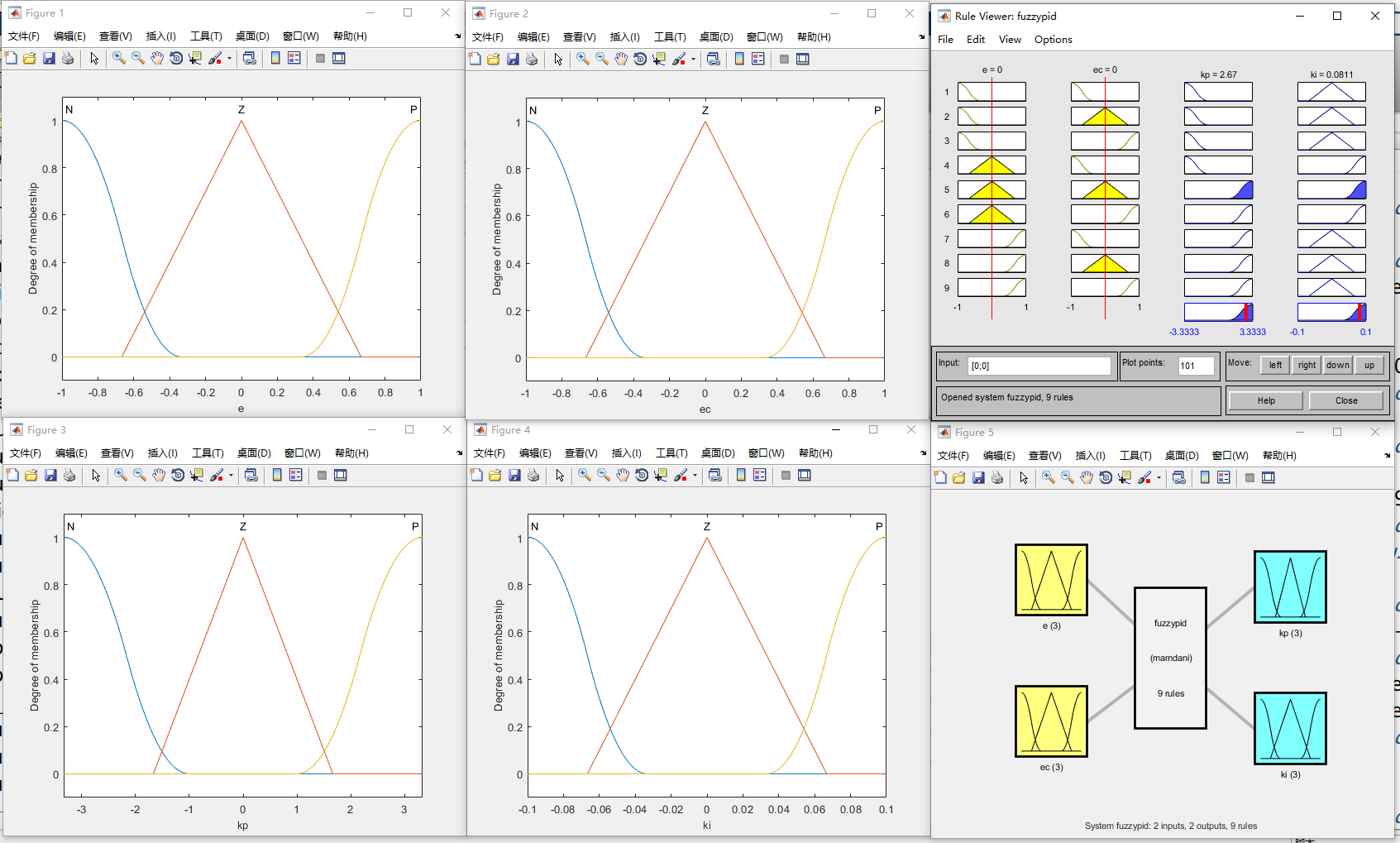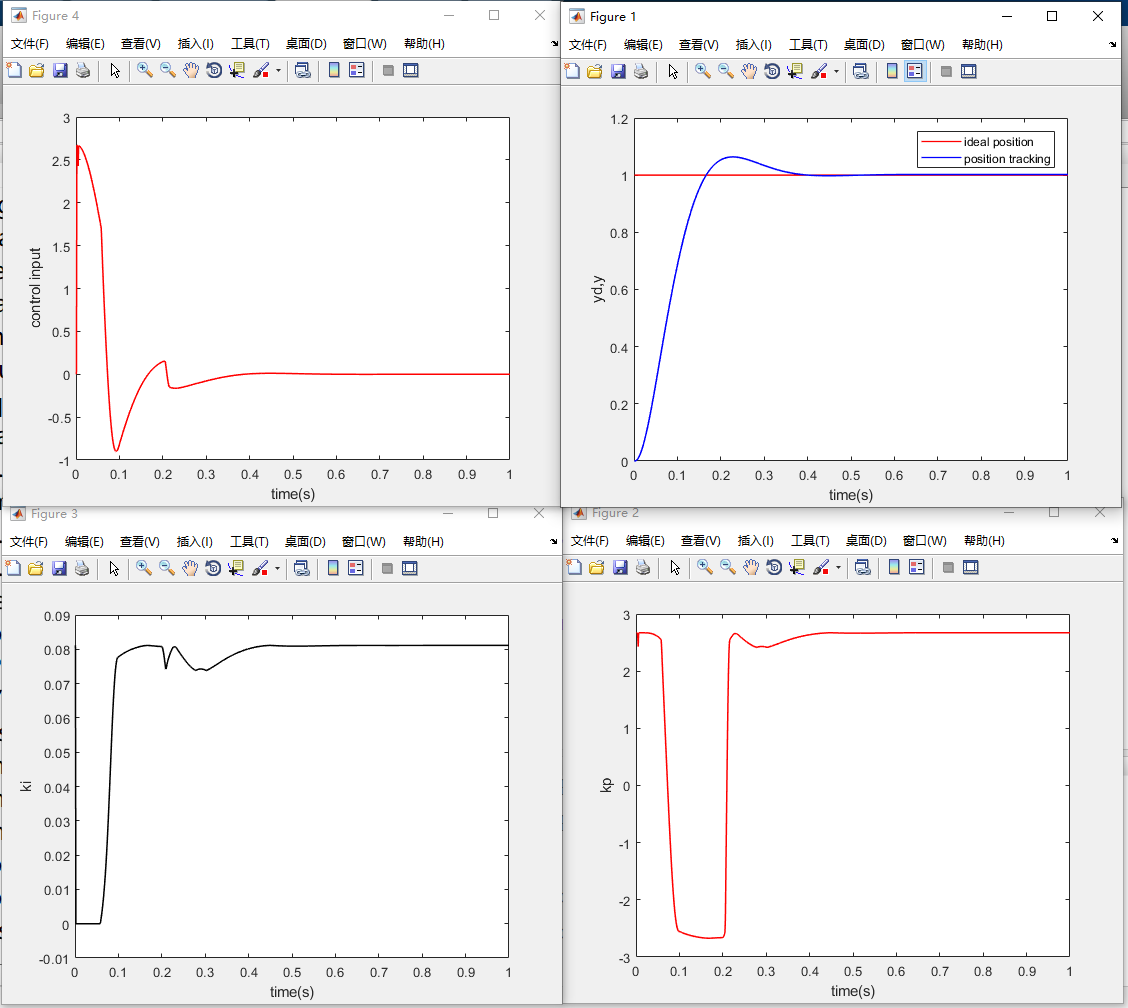展开全文matlab pid
• 比例阀介绍 ​ 以Parker公司的VSO® LOWPRO微型比例阀为例，该比例阀具有体积小，功耗低等优势。...下图是该比例阀的主要性能参数，与软件控制相关的主要有操作压力，控制滞环和相应时间等。 比例阀理论模型 ​ 比例
比例阀介绍
​	以Parker公司的VSO® LOWPRO微型比例阀为例，该比例阀具有体积小，功耗低等优势。
​	下图是在12V供电，1.7bar的压力条件下，比例阀的流量与控制电流之间的关系曲线，可以发现比例阀的流量控制具有滞环的特点。​	下图则是比例阀的流量与入口压力之间的关系曲线， 从图中可以看出流量随着压力的增大，但是增长速度在减慢，与平方根关系曲线接近，这和阀的理论模型是符合的。下图是该比例阀的主要性能参数，与软件控制相关的主要有操作压力，控制滞环和相应时间等。比例阀理论模型
​	比例阀的输出流量与气体性质，有效通气面积，阀门前后两端的气压压差有关，呼吸机中通过比例阀的气体绝大部分为氧气和空气（$N_2,O_2$），均为双原子气体分子，气体动力学性质基本相同，在模型中可以不用考虑气体自身性质的影响。因此可以得到比例阀的理论数学模型如下：
$Q=k(v)A\sqrt{\frac{g}{5}} \sqrt{\Delta P}H(s)\\ H(s)=\frac{1}{Ts+1}$
​    其中：Q为流过比例阀的流量（L/s），A为比例阀开度到最大时的等效通气面积($cm^2$)，$\Delta P$ 为比例阀前后的气压差($cmH_2O$)。H(s) 为惯性环节，用来表示比例阀的动态特性，k 为一个系数，与比例阀的驱动电压有关，表示在驱动电压的控制下比例阀的开度情况。g为重力加速度($m/s^2$)。
​	将datasheet中的直径参数带入到该理论模型中，画出流量与压力之间的理论曲线如下图所示，将理论模型的曲线与datasheet中给出的曲线对比，当直径较小时，理论模型与实际较接近，当直径较大时，理论流量要偏大。比例阀流量控制测试
比例阀驱动电路
​	下图是一个比例阀驱动电路，驱动电路的输入为来自控制芯片的使能信号和PWM脉冲信号。通过控制PWM脉冲的占空比就可以控制比例阀端口的驱动电流，从而达到控制流量的目的。比例阀流量控制测试方法
​	比例阀的驱动脉冲信号的占空比对应程序中的一个数字量（PWM比较寄存器值），通过控制这个数字量就能够控制比例阀的输入端口电流，从而控制流量。
​	将呼吸机的吸气管道接到PF300，同时将呼气管堵住，然后接入氧气源（氧气瓶）。使用仿真器连接呼吸机控制器通过在线仿真的方式，实时给定对应PWM模块的比较寄存器值，记录比较寄存器的值和PF300以及呼吸机内部测量得到的流量数据等。
​	在不同的气源压力下按照如前所述的流量控制测试，下面给出一组在初始气源压力为290kpa时的测试数据：

CMPA
DUTY
O2 Supply Pressure(kpa)
FLOW in PFC3000(L/min)
FLOW in Sensor(L/min)

0
0
287
0
0

300
4
287
0
0

600
8
287
0
0

900
12
287
0
0

1200
16
287
0
0

1500
20
287
0
0

1800
24
287
0
0

2100
28
286
0
0

2400
32
286
0
0.1

2700
36
284
1.3
1.4

3000
40
283
3.5
3.5

3300
44
281
5.9
6

3600
48
280
8.4
8.4

3900
52
279
11
11.1

4200
56
278
13.9
13.9

4500
60
278
16.3
16.4

4800
64
277
19.1
19.2

5100
68
276
22.3
22.6

5400
72
275
25.5
25.8

5700
76
274
28.8
29

6000
80
274
31.7
31.7

6300
84
273
34.5
34

6600
88
272
37.1
37.3

6900
92
272
39
39.1

7200
96
272
39
39

7500
100
272
39
39

7200
96
272
39
39

6900
92
272
38.7
38.4

6600
88
274
37
37

6300
84
274
34.9
35

6000
80
276
32.6
32.8

5700
76
276
29.9
30.3

5400
72
277
27
27.4

5100
68
278
23.8
24.3

4800
64
279
20.7
21.1

4500
60
280
17.8
18

4200
56
281
15
15.1

3900
52
282
12.2
12.2

3600
48
283
9.5
9.5

3300
44
283
6.9
6.9

3000
40
285
4.4
4.4

2700
36
287
2.2
2.1

2400
32
289
0
0.3

2100
28
289
0
0

1800
24
289
0
0

1500
20
289
0
0

1200
16
289
0
0

900
12
289
0
0

600
8
289
0
0

300
4
289
0
0

0
0
289
0
0

比例阀测试数据分析
​	根据记录的数据，可以画出程序内PWM信号占空比与实际流量之间的关系，下图是290kpa下测试得到的曲线，从曲线中可以看出，比例阀的控制存在滞环，但是滞环的影响较小，相同流量情况下的占空比差不超过5%。从曲线还可以看出，当驱动信号占空比低于一个开启阀值时，比例阀完全关闭，没有流量。高于一个饱和值时，比例阀完全打开，流量也基本不再变化。而在这中间，流量数据与驱动信号的占空比基本呈线性关系。​	从理论模型中可以知道，流过比例阀的流量还与比例阀两端的气压差有关，由于实际使用中比例阀出口端的压力相较于入口端的压力很小，因此出口端的压力变化可以忽略，仅考虑入口端的气源压力的影响。下图是在不同气源压力下进行测试得到数据绘制的曲线（仅考虑阀由闭到开的过程）。从图中可以看出，在气源压力不同时，流量随驱动信号占空比变化曲线的偏移是很小的。这可能与气源压力的变化范围，流量变化范围比较小有关。​
​	考虑到实际测试中在一般使用范围内，气源压力对流量控制的影响较小，可以选择一个实际应用中气源压力的典型值400kpa，来拟合得到驱动信号占空比与流量之间的关系。通过线性拟合，可以得到驱动信号占空比和流量之间的关系如下：
$Q = \begin{cases} 0, & \text{if } D<34\\ 0.676D - 22.976, & \text{if } 3491 \end{cases}$
上式中Q表示流量，D表示驱动信号的占空比。
比例阀模型在控制中的应用
1. 在流量控制闭环中引入前馈量
​	在得到了占空比与流量的关系之后，可以根据目标流量值反推出控制器应该给出的控制信号占空比。将该控制量作为流量控制环路的前馈项，可以提高系统的动态响应性能，降低对PI控制器的带宽要求和饱和风险，提高控制稳定性。引入前馈项后的比例阀控制框图如下图所示：
​	前馈控制用于确定基本的控制量级别，来得快且直接，反馈控制用于误差修正，能确保稳和准，两者配合则可获得各自优点。如果前馈控制做得足够准的话，反馈控制是可以去掉的，但是实际很少这样做，因为系统是不断变化的，需要反馈保证稳定性。2. 比例阀控制PI参数计算
​	将实验得到的比例阀的模型，带入到上述流量控制系统中，同时考虑实际控制中的延时环节，可以得到流量反馈控制的传递函数模型。根据模型，按照所需要的流量控制带宽和相角裕度，可以计算出PI控制器的参数值。可以按照如下方式计算比例阀的PI控制参数，可在matlab等软件中完成如下计算过程：

比例阀控制器传递函数:
$G_c(s)=k_p+\frac{k_i}{s}$

控制器延时传递函数:
$G_{d1}(s)=e^{-\frac{3}{2}T_c s}$
其中$T_c$ 为控制周期。

比例阀传递函数
$G_{valve}(s)=k_v \frac{1}{T_v s+1}$
其中$k_v, T_v$ 分别为比例阀模型中的系数和时间常数。

采样延时传递函数
$G_{d2}=\frac{1}{T_{s}s+1}$
其中$T_s$ 表示采样延时。

开环传递函数
$G_{op}(s)=G_{c}(s) G_{d1}(s)G_{d2}(s)G_{valve}(s)$

闭环传递函数
$G_{cl}(s)=\frac{G_c(s)G_{d1}(s)G_{valve}(s)}{1+G_{op}(s)}$

根据系统的动态响应速度，超调量与稳定性要求给出需要的控制带宽 $f_c$ 和相角裕度 $M$ 。

根据开环传函在截止频率处的模值为1，以及相角裕度的定义列出如下方程：
$\begin{cases} \lVert G_{op}(2\pi f_ci)\rVert =1 \\ \angle G_{op}(2\pi f_ci)+180=M \end{cases}$
根据上述方程求解出PI控制器中的$k_p$ ，$k_i$ 参数。

PI参数离散化，增量式PI控制器的表达式如下：
KaTeX parse error: No such environment: align at position 8:
\begin{̲a̲l̲i̲g̲n̲}̲
u(k) &=u(k-1)…
其中$u(k)$ 表示k时刻的控制器输出，$e(k)$ 表示k时刻的给定和反馈之间的误差。离散化后的系数和PI参数有如下关系：
KaTeX parse error: No such environment: align at position 8:
\begin{̲a̲l̲i̲g̲n̲}̲
\alpha &= k_p …
其中Ts为控制周期。

验算，测试。
这是根据NNP所用比例阀测试数据，选择控制带宽50hz，相角裕度80度计算得到PI参数后，做出比例阀流量控制系统的闭环传递函数波特图以及阶跃响应曲线。
​	从波特图可以看出闭环控制带宽为50hz，从系统阶跃响应可以看出系统的上升时间为7ms,超调小于10%.PI参数自动计算代码
控制系统PI参数设计和计算源码：
https://github.com/RanFang66/ControlSystemDesign


展开全文c语言 pid matlab
• ## 数字PI控制器

千次阅读 2015-04-18 20:44:59
数字PI控制器是控制系统中常用的简单有效的控制算法， 用C语言实现该算法，使用与各类嵌入式控制系统中
最近项目需要用到PI控制器，于是参考网上的一些资料，尝试着写了一下，将它记录下来以备后用。

PID公式：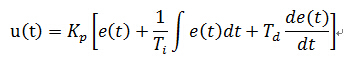Ti，Td分别为积分，微分常数，只取PI部分：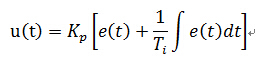根据采样周期T离散化，t=nT,用n表示，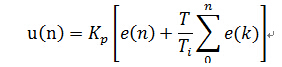可得增量式：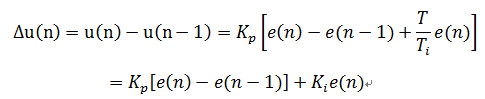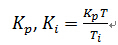分别为比例，积分系数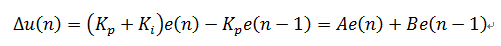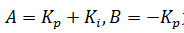为计算系数

最终输出为：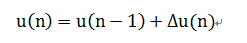写代码时，定义一个结构体来存放各个参数，
程序设计流程为：

本次采样输入→计算本次误差→计算输出增量→更新数据为下次做准备
附源码：
/************声明和定义，通常放在头文件中********/
typedef struct
{
int SetValue;        //设置值
int An;			//当前误差e(n)的系数，=kp+ki
int Bn_1;			//前一项误差e(n-1)的系数，= -kp
int Limit;			//限制最大最小值
int Errorn_1;		//e(n-1)
int DeltaUn;		//输出增量△u(n);
int Un_output;	//输出值 u(n)
}PI_Typedef;

void PI_StructInit(PI_Typedef *PI_Struct,int Kp,int Ki,int limit);
void PI_Controller(PI_Typedef *PI_Struct,int NewInput,int *Output);
/*****************************************************************/

void PI_StructInit(PI_Typedef *PI_Struct,int Kp,int Ki,int limit)
{
PI_Struct->SetValue = 0;
PI_Struct->An = Kp+Ki;
PI_Struct->Bn_1 = -Kp;
PI_Struct->Limit = limit;
PI_Struct->DeltaUn = 0;
PI_Struct->Errorn_1 = 0;
}

void PI_Controller(PI_Typedef *PI_Struct,int NewInput,int *Output)
{
int Errorn =0;

Errorn = PI_Struct->SetValue - NewInput;//计算e(n)

PI_Struct->DeltaUn = (PI_Struct->An)*Errorn+(PI_Struct->Bn_1)*(PI_Struct->Errorn_1);//计算增量
PI_Struct->Errorn_1 = Errorn;//保存误差e(n)作为下一次计算的e(n-1)
//限制增量的范围
if(PI_Struct->DeltaUn > PI_Struct->Limit)
{PI_Struct->DeltaUn = PI_Struct->Limit;}
else if(PI_Struct->DeltaUn < -PI_Struct->Limit)
{PI_Struct->DeltaUn = -PI_Struct->Limit;}

PI_Struct->Un_output += PI_Struct->DeltaUn; //计算输出u(n)=u(n-1)+△u(n),并保存作下一次运算

*Output = PI_Struct->Un_output;
}

wind

2015,04,18


展开全文PI算法 c语言
• 感应（异步）电机磁场定向控制电流环PI控制参数设计 目录电机控制系列文章前言一、速度环开环传递函数二、速度环开环传递函数零极点配置三、速度环闭环性能四、速度滤波器的影响总结 前言 大家在做感应（异步）...matlab simulink
• 目录前言一、并联型PI与串联型PI二、被控对象模型三、电流环闭环传递函数四、电流环PI参数设计五、计算延时、PWM采样延时、滤波器的影响1、计算延时2、PWM采样延时总结 前言 大家在做感应（异步）电机磁场定向控制...matlab simulink
• 3 PI控制器参数整定 3.1从PMSM电机的数学模型出发。 dq 轴 电压方程： dq 轴 轴磁链方程： dq 轴 转矩方程： dq 轴 运动方程： 分析上述方程，如果我们能够控制 id=0 那么电压...永磁同步电机
• 一般伺服驱动都具备位置、速度、和电流控制，框图如下图所示： 伺服驱动的电流环通常采用矢量控制方法，在很多电机控制书籍中都有很详细的介绍，本文结合具体实例，简单介绍其设计步骤。 一、电机状态方程 以...
• 通过 Matlab仿真和水箱液位控制系统的实际操作实验,对分数阶 PIλ控制器、整数阶 PI控制器和常规 Ziegler-Nichols参数 整定方法进行比较,说明了本文设计方法的有效性和分数阶 PIλ控制器的优越性.
• Waveshare给您介绍一款树莓派家族的新成员，Raspberry Pi Pico 是一款低成本，高性能的微控制器开发板，具有灵活数字接口。 硬件上，采用Raspberry Pi 官方自主研发的 RP2040 微控制器芯片，搭载了ARM Cortex M0 + ...单片机 树莓派
• 光伏并网逆变采用双闭环控制模式，输出电压正常，输出电流不稳定，是内环PI参数设置有问题，还是外环设置有问题，PI参数该如何调节呢。附上结果图片。 ...
• 这是对永磁同步电机矢量控制PI调节器参数的计算，先通过计算内环电流调节器的PI，再调节外环转速环PI调节器的参数。计算出来的数据对永磁同步电机矢量控制的效果非常好，这哥方法也很有用。
• 主要包括三个部分：转速环PI调节，电流环PI调节，SVPWM算法等。 主要参数计算 3.1 转速环ADRC理论分析 请参考赠送的文献或者硕士论文。谢谢。 3.2 电流环PI参数理论分析 3.3 电流环PI参数...永磁同步电机 SVPWM
• 1 截止频率 将内环看作一个采样环节，对外环给定信号进行采样。...在实际应用中比如PFC或者并网逆变器双环控制中，电流内环控制器截止频率一般是开关频率的1/10，电压外环的截止频率一般取100HZ以内 2 开...
• 本文主要详细分析PIDPIDPID控制器各个环节的作用以及参数整定的一般方法。 PIDPIDPID控制器各环节的作用： PPP：误差的比例项(现在)，比例项的引入，时域上来讲可以增加了系统的开环增益和自然频率，使控制系统的...频域分析
• 目前，常用的解耦设计方法，有前馈解耦、偏差解耦以及内模控制。 采用前馈解耦方法 由电机在旋转坐标系下的数学模型可以得到uqu_quq​和udu_dud​表达式： 对其取拉氏变换，得到 由此可以画出电机模型的传递函数：...永磁同步电机 前馈解耦
• 矢量控制系统是以控制电机的定子电流来控制转矩，如果不能有效控制定子电流，则无法有效控制其转矩。添加一个电流内环，可以使电机在负载波动时，动态调节定子电流，进而匹配电磁转矩，加快系统的动态响应速度。 ...永磁同步电机
• ## RBF+PID参数自整定控制器设计

万次阅读 热门讨论 2018-06-30 00:05:13pid
• 针对上述控制结构图，利用matlab整定参数。 %% Tuning Control Systems with SYSTUNE % systuneWorkflowExample.m G = linsys; C0 = tunablePID('C','pi'); % tunable PI a = realp('a',1); % 参数化 F0 = tf(a,[1 ...
• 1.什么是滞后补偿以及和PI控制的联系 2.PI控制对系统的影响 3.如何设计一个运动控制系统 4.抗积分饱和的方法 5.一个实例 1.什么是超前补偿以及和PI控制的联系 上一篇文章提到了超前补偿，超前这个词的含义...算法
• 这一节我们将对上一节的Buck电路进行闭环控制，控制方法采用最经典的PI控制器。 目标电压为15V。 电路的参数如下： PI的传递函数如下： 通过频率设计设计带宽为278rad/s，相位裕量为60°。则可以...
• 本篇文章首发于公众号：无人机系统技术。更多无人机技术相关文章请关注此公众号。...接下来的几篇内容将以多旋翼飞行器为例，介绍PID控制方法在控制器设计中的应用，还将以px4开源软件为例说明控制器设计的具体实...无人机 PID 入门
• 目录系统模型及参数控制性能目标PID概述比例控制PI 控制器PID 控制器 系统模型及参数 前面教程已经得出了定速巡航系统的传递函数模型： P(s)=V(s)U(s)=1ms+b[m/sN] P(s) = \frac{V(s)}{U(s)} = \frac{1}{ms+b} \...MATLAB PID
• 文章目录单回路控制系统整定参数整定要求常用整定方法理论计算方法理论计算整定法工程整定方法经验法(试凑法)临界比例带法(边界稳定法)衰减曲线法响应曲线法(动态特性参数法) 单回路控制系统整定 参数整定要求 通过...
• 模糊PI控制器由常规PI控制和模糊推理两部分组成，模糊推理部分的输入是偏差E和偏差变化率EC，输出量是、。PI参数模糊自整定就是找出PI参数和，之间的模糊关系，在运行中不断检测和，根据模糊控制原理来对三个参数...PID 模糊PID
• 为了使得开关电源有更好的稳态性能和动态性能，需要针对...本文简述了补偿的过程，以Buck开关电源为例，对未补偿系统进行分析，并设计补偿网络，最后对补偿后系统进行分析。经过分析，补偿后的系统性能优于补偿前系统。开关电源
• 在PID控制器的诸多经典参数整算法中，绝大多数的算法都是在带有时间延迟的一阶模型（FOPDT）的基础上提出的，模型的一般形式为： 主要是因为大部分过程控制的受控对象模型的响应曲线和一阶模型的响应较为类似，可以...
• 模拟速度控制器参数设置（走马观花的灌输）： 转矩调整器有延迟 直流驱动功率放大器 Or   电流控制器 由拉普拉斯变换可以得到下面电流控制器闭环框图： 然后会得到传递函数 在实践中一般将...
• [控制理论] （1） 二阶系统的滑模控制器设计[控制理论] （1） 二阶系统的滑模控制器设计1 前言 [控制理论] （1） 二阶系统的滑模控制器设计 本博客需要一些现代控制理论中Lyapunov稳定性的一些理论知识。 前文对......